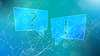# TechTopics No. 131

Heat generation estimation for SIEBREAK™ metal-enclosed interrupter switchgear

Siemens is often asked to provide estimated heat generation data for our equipment. This issue of TechTopics provides information that allows calculation of approximate heat generated by SIEBREAK metal-enclosed interrupter switchgear equipment under assumed loading conditions.

The heat generation data given in the tables for fuses assumes class E fuses, whether current-limiting or expulsion type (as in the relevant tables), assuming that the continuous current through the fuse is 80 percent of the E-rating of the fuse.

For applications which differ from these assumptions, convert the heat generation shown for the typical currents to that for the specific application by the ratio of the squares of the currents.

To estimate the heat generated under actual loading conditions, determine the component heat generation for each of the components indicated in the table, with adjustments if necessary for differing currents. Space heater load should be assumed to be continuous.

For simplicity, assume that main bus loading in each vertical section is equal to the total estimated load of the switchgear lineup, and adjust the heat generated for each vertical section by the square of the percentage of total estimated load to rated main bus current. While this is seldom correct, the overstatement of the heat generation is not often significant.

The tables which follow show total heat generation for the building block configurations described, and serve as the basis of calculations for an actual switchgear configuration involved with a project.

Since the heat generation value given is based on rated current for assemblies, the value of heat generation must be adjusted to reflect the actual current in the application.

Heat generated by current transformers is ignored as it is usually insignificant, and varies according to the current transformer (CT) ratio as well as the loading.

The heat generation of control power transformers (CPTs) is highly variable, but if considered, should be estimated at about four percent of the VA rating of the transformer, e.g., for a 15 kVA CPT, estimate 600 W heat generation at full load.

Heat generation by relays and meters is generally ignored and is usually not significant in metal-enclosed interrupter switchgear. If relays and meters are included, 50 W is a very conservative estimate of heat generation by microprocessor relays or power meters.

If heat generation is desired in BTU/ hour, the conversion factor is:

watts X 3.415179 = BTU/hour.

Heat generation for typical section configurations

Heat generation by fuses

Space heaters, when provided, are either continuously energized, or alternatively, controlled by a nonadjustable thermostat set to keep the space heaters “on” when the temperature inside the section is 104 °F (40 °C) or lower. Hence, space heater load is assumed to be continuous. The purpose of space heaters is to prevent condensation, and this is not limited by the absolute temperature. In an air-conditioned room, the heaters would be energized continuously.

Example calculations

Now we have the heat generation estimates for the various building blocks. Several examples of calculations for specific switchgear assemblies follow. For each example, the heat generation in the assembly is adjusted for the actual current, by the ratio of the squares of actual current versus rated current. For fused switches, actual current is assumed to be 80 percent of the E-rating of the fuses. The heat generation for fuses is not adjusted for actual current.

Example one: 600 A fused switch, single unit, with 300E current-limiting fuses

Example two: 600 A fused selector switch, single unit, with 200E expulsion fuses

Example three: Lineup with 600 A LIS main switch (600E CL fuses), transition section, two 600 A fused LIS feeder switches (400E and 200E fuses), 600 A main bus, outdoor

In example 3, the heat generation for the main bus and transition was used as though they were carrying their full 600 A continuous current rating. The heat generation associated with the bus in this example is 451 W. A more rigorous calculation would use 480 A for two units and the transition, and 160 A for the last unit. Then, the heat generation for the bus would be:

This would result in 451 W – 246 W = 205 W reduction in heat generation. This is a comparatively small reduction (205 W is 5.8 percent of the total heat of 3,510 W with space heaters, and only 10.7 percent of the total heat of 1,910 W if heaters are not involved) and explains why the reduction in current as the units get farther from the source is usually ignored.